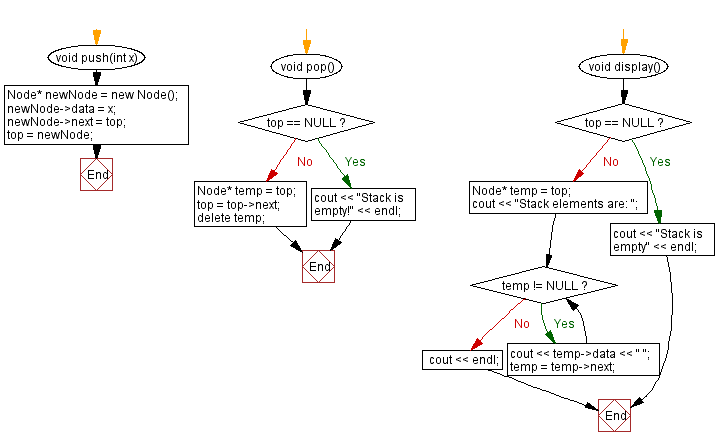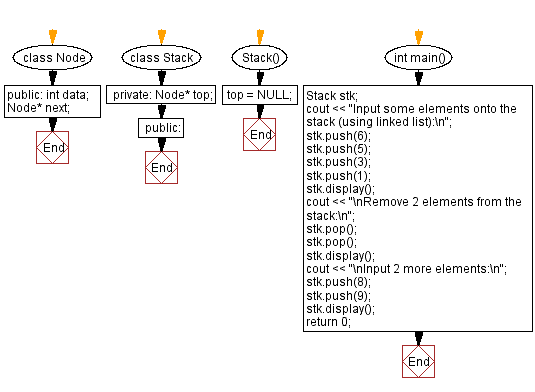﻿ C++ Implement a stack using a linked list with push, pop

# C++ Stack Exercises: Implement a stack using a linked list with push, pop

## C++ Stack: Exercise-16 with Solution

Write a C++ program to implement a stack using a linked list with push, pop operations.

Test Data:
Input some elements onto the stack (using linked list):
Stack elements are: 1 3 5 6
Remove 2 elements from the stack:
Stack elements are: 5 6

Sample Solution:

C++ Code:

``````#include <iostream>

using namespace std;

class Node {
public:
int data;
Node* next;
};

class Stack {
private:
Node* top;
public:
Stack() {
top = NULL;
}
void push(int x) {
Node* newNode = new Node();
newNode->data = x;
newNode->next = top;
top = newNode;
}
void pop() {
if (top == NULL) {
cout << "Stack is empty!" << endl;
return;
}
Node* temp = top;
top = top->next;
delete temp;
}
void display() {
if (top == NULL) {
cout << "Stack is empty" << endl;
return;
}
Node* temp = top;
cout << "Stack elements are: ";
while (temp != NULL) {
cout << temp->data << " ";
temp = temp->next;
}
cout << endl;
}
};

int main() {
Stack stk;
cout << "Input some elements onto the stack (using linked list):\n";
stk.push(6);
stk.push(5);
stk.push(3);
stk.push(1);
stk.display();
cout << "\nRemove 2 elements from the stack:\n";
stk.pop();
stk.pop();
stk.display();
cout << "\nInput 2 more elements:\n";
stk.push(8);
stk.push(9);
stk.display();
return 0;
}
``````

Sample Output:

```Input some elements onto the stack (using linked list):
Stack elements are: 1 3 5 6

Remove 2 elements from the stack:
Stack elements are: 5 6

Input 2 more elements:
Stack elements are: 9 8 5 6
```

Flowchart:CPP Code Editor: